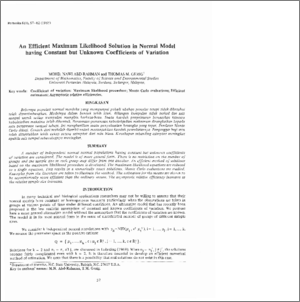# An Efficient Maximum Likelihood Solution in Normal Model having Constant but Unknown Coefficients of Variation

## Citation

Gerig, Thomas M. and Abdul Rahman, Mohd Nawi (1983) An Efficient Maximum Likelihood Solution in Normal Model having Constant but Unknown Coefficients of Variation. Pertanika, 6 (1). pp. 57-62.

## Abstract

A number of independent normal normal populations having constant but unknown coefficienls of variation are considered. The model is of more general form. There is no restriction on the number of groups and the sample size in each group may differ from one another. An efficient method of solutions based on the maximum likelihood procedure is developed. The maximum likelihood equations are reduced to a single equation. This results in a numerically exact solutions. Monte Carlo evaluations are studied. Examples from the literature are taken to illustrate the method. The estimators for the means are shown to be asymptotically more efficient than the ordinary means. The asymptotic relative efficiency increases as the relative sample size increases.Preview
PDF
An_Efficient_Maximum_Likelihood_Solution_in_Normal_Model.pdfView Item